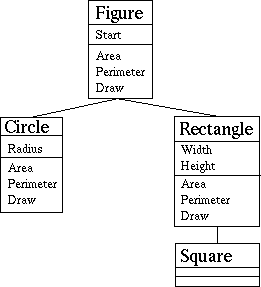# Section 7.2 - Object-Oriented Programming in Ada: Inheritance

One of the major features of OO programming is its use of inheritance. In 1995 facilities were added to Ada to easily support inheritance.

Inheritance lets us define new types as extensions of existing types; these new types inherit all the operations of the types they extend. The new types are termed `children' or `derived types', while the types extended are usually called `parents' or `base types.' Inherited operations can be overridden with new definitions of those operations. Derived types can also add new operations that apply only to them and not their base types.

In Ada 95 terminology, types that can have parents or children are termed ``tagged types'', and have the keyword ``tagged'' as part of their definition.

This is probably best shown through an example. Let's imagine that we're writing a program that must display many different kinds of geometric figures, such as circles, rectangles, and squares. We could create different types to represent the figures, but clearly these different kinds of figures share a number of things in common. In particular, all of these things can be drawn, and all of them have areas. We could create a package called "Figures" to define these different types, and we could define a type called "Figure" to represent any of these different kinds of figures (circles, rectangles, or squares). We could then say that circles and rectangles are a kind of figure, and squares are a kind of rectangle. A given type includes all of the record components specific to it, plus all the record components of its ancestors, so a square has all the information a rectangle would.

We'll also need to define some operations on these types, such as "Draw", "Area", and "Perimeter". For example, function Area will determine the figure's surface area and return it as a Float. If we don't redefine a subprogram for a given type, the closest ancestor's defined subprogram will be used. For example, if we don't redefine subprogram "Area" for a Square, the "Area" subprogram defined for Rectangle will be used. That means that "Area" is still a perfectly valid subprogram for a Square.

Here's a graphical representation these interconnected types; the larger boxes represent the tagged types, showing the tagged type's name, additional data content, and new or overridden operations. The connections show the an inheritance relationship, with the parent type above the child type.We will also need a "Point" type to store the starting X and Y coordinates for each figure. To keep things short we'll just define the "Point" type in the same package. Since every figure needs a starting position, we'll put a starting position (of type Point) in the Figure type. Here's an example of how to write this in Ada:

```package Figures is
-- Package to demonstrate Object Orientation.

type Point is
record
X, Y: Float;
end record;

type Figure is tagged
record
Start : Point;
end record;
function Area  (F: Figure) return Float;
function Perimeter (F:Figure) return Float;
procedure Draw (F: Figure);

type Circle is new Figure with
record
end record;
function  Area (C: Circle) return Float;
function  Perimeter (C: Circle) return Float;
procedure Draw (C: Circle);

type Rectangle is new Figure with
record
Width: Float;
Height: Float;
end record;
function Area (R: Rectangle) return Float;
function Perimeter (R: Rectangle) return Float;
procedure Draw (R: Rectangle);

type Square is new Rectangle with null record;

end Figures;
```Quiz:

How many record components and subprograms are defined in total for type Square in package Figures?

You may also:

David A. Wheeler (dwheeler@dwheeler.com)

The master copy of this file is at "http://www.adahome.com/Tutorials/Lovelace/s7s2.htm".